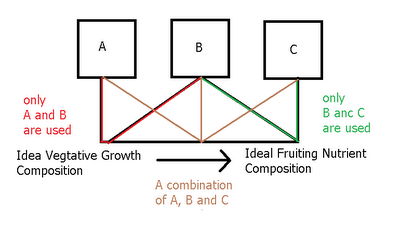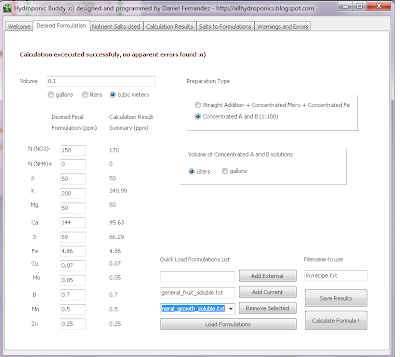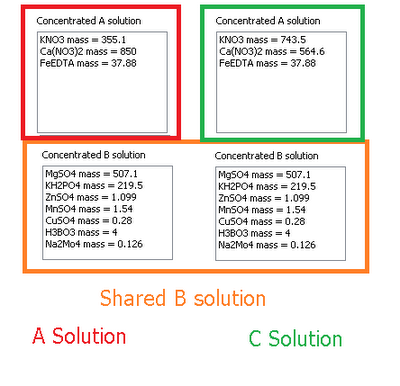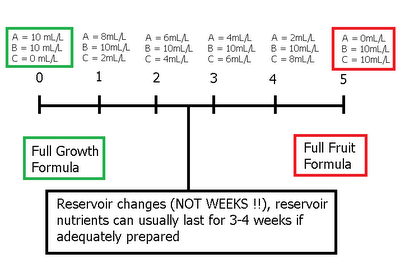# Preparing A, B and C (three part) Concentrated Nutrient Solutions, a Tutorial for my Hydroponic Nutrient Calculator

What is the difference between a 2 and a 3 part formulation ? What we need to understand here is that plants usually have different needs through their whole life cycle and therefore they require different nutrient ratios as they grow older and face different stages of their development. With a two part concentrated nutrient based formulation we can only vary this ratios in a certain way and doing any variations outside this scope will not be possible. With the default approach in my calculator you can vary nutrient ratios by varying the "desired formulation" every time you prepare your reservoir again (since most nutrients are added directly) but with the A and B concentrated solutions you are bound to "stick" to a certain set of concentration values for each nutrient.
--
The solution to this problem is quite simple and this is what most commercial fertilizer sellers have come up with. You have two solutions with the same salts but varied nutrient ratios and a third solution that remains constant. What we have then is two possibilities of final compositions A-B and C-B and a whole new possibility for nutrient ratios by combining A-B-C. What is done most of the time is that A-B becomes an ideal formulation for vegetative growth while C-B is an ideal formulation for fruiting. When you start to grow you generally do so with A-B and then you move towards C-B by increasing C and decreasing A as reservoir changes happen.

In my program this sort of solution scheme is easily achieved. What you need to do is simply to have two desired formulations, one for ideal growth and one for ideal flowering and then you just need to make two sets of A and B calculations where B is shared amongst the two. To do this we will first load the general_growth_soluble.txt and general_fruit_soluble.txt desired formulations as indicated on the tutorial for the preparation of A and B formulations (you can download them here and here). After loading the general_growth_soluble.txt composition you should have the formulation composition displayed below.
--
Then follow the same procedure outlined on the "A and B solution tutorial" to arrive to the salt-weight compositions of the A and B formulations (save the results using the "save results" button) . Now load the general_fruit_soluble.txt formulation and repeat the calculation, also saving your results. A summary of the results of both calculations is shown in the image presented below. You will notice that both calculations share the same weights for the B solution (meaning they use the same B solution) while the composition of the A solution changes. What you have now is a basic three part formulation. You could now think about a regime to change from an A-B growth solution to the C-B fruiting solution in the amount it takes your plants to bear fruit.
--
For example, if your plants took aproximately 5 reservoir changes you would then use different quantities of A and C to go from a 100% A feed to a 100% C feed during that time. A timeline if it takes your plants 5 reservoir changes to get to fruiting is shown below. Note how in the beginning we use 10mL of A per liter and then in the end we use 10mL of C per liter, meaning that we have done a full gradual change from a growth to a fruiting formulation.
--
As you see from the above, you can easily use my hydroponic calculator to prepare your own three part concentrated nutrient blends which you can use to build your own nutrient schedule to go from vegeative growth to fruiting. However if you are currently using a fixed composition of a three part commercial nutrient blend then you can easily prepare an A and B solution to replace it, three part nutrients are ONLY needed when you want to do a varying schedule like the one introduced above, using three part nutrients without doing this is simply an overcomplication since a fixed composition can be prepared using just two custom concentrated solutions.

### 4 Responses

1.Thank you very much. Your articles make many ( Whys ) in & of hydroponics very clear.
2.Do you know the formula of the Super Nutrients: http://www.abfertilizer.ca/products.html I'd like to mix my own nutrients from salt, but don't know formula to follow. thanks.
3.Can you please explain to me why some use25ml of A& 25ml of B to 10 L of water and some says use 100 ml A &100ml B to 10 L of water. Which formula should I use. I’m a very beginner gardener trying to do hydroponic lettuce n some chilli plants. Thanks Helen
•Thanks for commenting! Solutions can be prepared with different dilution ratios, so a solution that's more concentrated will require less to be added per liter while a less concentrated solution will require more to be added. Follow the manufacturer's instructions if you're using a commercial solution.